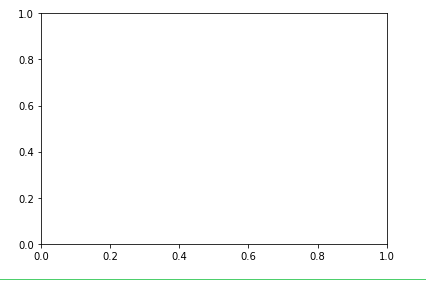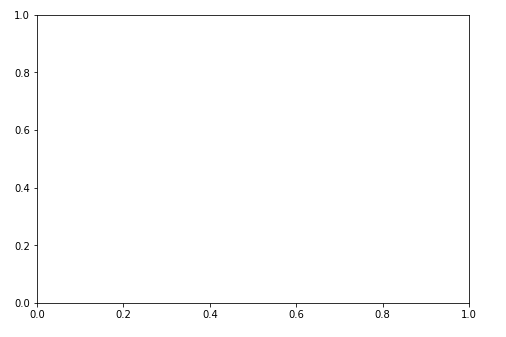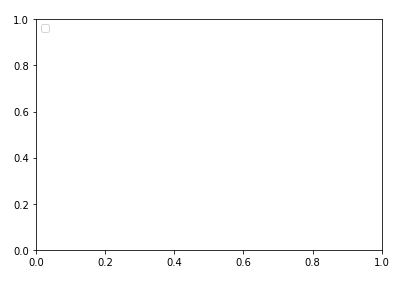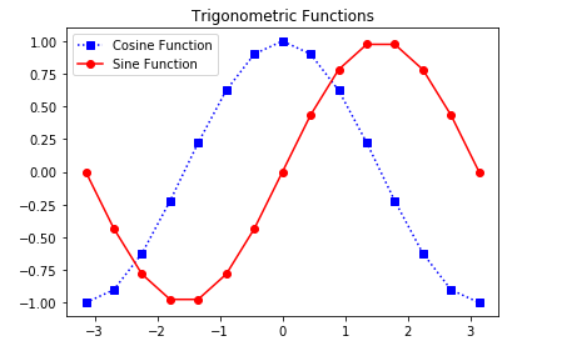Related Articles

# Matplotlib – Axes Class

• Last Updated : 27 May, 2020

Matplotlib is one of the Python packages which is used for data visualization. You can use the NumPy library to convert data into an array and numerical mathematics extension of Python. Matplotlib library is used for making 2D plots from data in arrays.

## Axes class

Axes is the most basic and flexible unit for creating sub-plots. Axes allow placement of plots at any location in the figure. A given figure can contain many axes, but a given axes object can only be in one figure. The axes contain two axis objects 2D as well as, three-axis objects in the case of 3D. Let’s look at some basic functions of this class.

### axes() function

`axes()` function creates axes object with argument, where argument is a list of 4 elements [left, bottom, width, height]. Let us now take a brief look to understand the axes() function.

Syntax :

`axes([left, bottom, width, height])`

Example:

 `import` `matplotlib.pyplot as plt ` ` `  ` `  `fig ``=` `plt.figure() ` ` `  `#[left, bottom, width, height] ` `ax ``=` `plt.axes([``0.1``, ``0.1``, ``0.8``, ``0.8``])  `

Output:Here in `axes([0.1, 0.1, 0.8, 0.8])`, the first ‘0.1’ refers to the distance between the left side axis and border of the figure window is 10%, of the total width of the figure window. The second ‘0.1’ refers to the distance between the bottom side axis and the border of the figure window is 10%, of the total height of the figure window. The first ‘0.8’ means the axes width from left to right is 80% and the latter ‘0.8’ means the axes height from the bottom to the top is 80%.

Alternatively, you can also add the axes object to the figure by calling the `add_axes()` method. It returns the axes object and adds axes at position [left, bottom, width, height] where all quantities are in fractions of figure width and height.

Syntax :

`add_axes([left, bottom, width, height])`

Example :

 `import` `matplotlib.pyplot as plt ` ` `  ` `  `fig ``=` `plt.figure() ` ` `  `#[left, bottom, width, height] ` `ax ``=` `fig.add_axes([``0``, ``0``, ``1``, ``1``]) `

Output:### ax.legend() function

Adding legend to the plot figure can be done by calling the legend() function of the axes class. It consists of three arguments.

Syntax :

`ax.legend(handles, labels, loc)`

Where labels refers to a sequence of string and handles, a sequence of Line2D or Patch instances, loc can be a string or an integer specifying the location of legend.

Example :

 `import` `matplotlib.pyplot as plt ` ` `  ` `  `fig ``=` `plt.figure() ` ` `  `#[left, bottom, width, height] ` `ax ``=` `plt.axes([``0.1``, ``0.1``, ``0.8``, ``0.8``])  ` ` `  `ax.legend(labels ``=` `(``'label1'``, ``'label2'``),  ` `          ``loc ``=` `'upper left'``) `

Output:### ax.plot() function

`plot()` function of the axes class plots the values of one array versus another as line or marker.

Syntax : plt.plot(X, Y, ‘CLM’)

Parameters:
X is x-axis.
Y is y-axis.
‘CLM’ stands for Color, Line and Marker.

Note: Line can be of different styles such as dotted line `(':')`, dashed line `('—')`, solid line `('-')` and many more.

Marker codes

Characters Description
‘.’ Point Marker
‘o’ Circle Marker
‘+’ Plus Marker
‘s’ Square Marker
‘D’ Diamond Marker
‘H’ Hexagon Marker

Example: The following example shows the graph of sine and cosine functions.

 `import` `matplotlib.pyplot as plt ` `import` `numpy as np ` ` `  ` `  `X ``=` `np.linspace(``-``np.pi, np.pi, ``15``) ` `C ``=` `np.cos(X) ` `S ``=` `np.sin(X) ` ` `  `# [left, bottom, width, height] ` `ax ``=` `plt.axes([``0.1``, ``0.1``, ``0.8``, ``0.8``])  ` ` `  `# 'bs:' mentions blue color, square  ` `# marker with dotted line. ` `ax1 ``=` `ax.plot(X, C, ``'bs:'``)  ` ` `  `#'ro-' mentions red color, circle  ` `# marker with solid line. ` `ax2 ``=` `ax.plot(X, S, ``'ro-'``)  ` ` `  `ax.legend(labels ``=` `(``'Cosine Function'``,  ` `                    ``'Sine Function'``),  ` `          ``loc ``=` `'upper left'``) ` ` `  `ax.set_title(``"Trigonometric Functions"``) ` ` `  `plt.show() `

Output:Attention geek! Strengthen your foundations with the Python Programming Foundation Course and learn the basics.

To begin with, your interview preparations Enhance your Data Structures concepts with the Python DS Course. And to begin with your Machine Learning Journey, join the Machine Learning – Basic Level Course

My Personal Notes arrow_drop_up
Recommended Articles
Page :